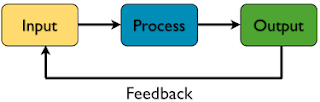Learn Input and Output in PythonINPUT & OUTPUT

Input is the input that you give to the python program. python program will process it and display the output results. Input, process and output are at the core of all computer python program.

Input Proses dan OutputIn this article, you will learn how to take input and display output to a text-based python program.

How To Take Input from Keyboard

Python is already providing input function () and raw_input () to take input from the keyboard.

Way of life:
name_variabel = input ( "A Text")
That is, the text that you fed from the keyboard will be recorded into variable_name.

Please you try an example below ...

# Take input
name = raw_input ( "What is your name:")
age = input ( "How old are you")

# Display output
print "Hello", the name, "age you are," age, "the"
result:

Program Python Input Output Simple

What is the difference input function () and raw_input ()?

Input function () is used to retrieve data values. While raw_input () to retrieve the text.

In Python3 simply use the input function (), because the function raw_input () are combined there.

How to Display Output

As you already know in the previous examples.

To display the output text, you use the function print ().

Example:

print "Hello World!"
print name_variabel
print "Join With", variabel

Displaying Variable and Text

In the above example you use a comma (,) to combine text and variables will be displayed.
``````
name = "FajarYusuf"
print "Hello",name
``````

Results:

Some say Hello and FajarYusuf available space as a separator, because you use a comma.

Watch Out…

Do not add parenthesis like this:
``````
name = "FajarYusuf"
print("Hello",name)
``````

Because it will be read as a tuple which produces a rich output like this:

Do not use parentheses when using a comma.

If you want to use parentheses, you must combine text and variable with a plus sign (+).

Example code:
``````
name = "FajarYusuf"
print("Hello " + name)``````

The result:

Using the Format function ()

Format function () will combine the contents of the variable text.

Example code:
``````
name = raw_input("Name:  ")
print "Hey {} Whatsapp ?".format(name)
``````

The result:i input Fajar Yusuf for Name

Sign {} will automatically be replaced in accordance with the value that you fed to the variable name.

More coding examples:
``````
her_name= raw_input("Her Name : ")
print "{} with {} is the best couple :)".format(your_name, her_name)
``````

The result:i input romeo for your name, and juliet for her name

Using String Formatting Old Ways

The incorporation of variable text and how long to use the percent symbol (%).

Example code:
``````
name = raw_input("Input name : ")
print "Hey Welcome %s" % name
``````

The result:i input romeo for input name

Signs% s will automatically be replaced with the value that you fed to the variable name.

Example code again:
``````
name = raw_input("Input name : ")
age = input("Input age : ")
tall = input("Input tall : ")
print "Hello %s, your age is %d years old and tall is %f cm" % (name, age, tall)
``````

Signs% s for text data types,% d for numbers (decimal), and% f for fractions.

Berlangganan update artikel terbaru via email: